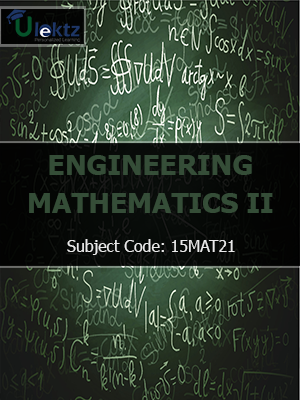•My WalletMy Order
•My Profile
•My Connections
•My Books
•My Videos
•My Tests
•My Calender
•My Messages
•My Shopping Cart
•My Orders
•Account Settings
•Help

# Book Details# Engineering Mathematics - II

 Course Code : 15MAT21 Author : uLektz University : Visvesvaraya Technological University, Karnataka (VTU) Regulation : 2015 Categories : Electronics & Communication Format :ePUB3 (DRM Protected) Type : eBook

FREE

Description :Engineering Mathematics - II of 15MAT21 covers the latest syllabus prescribed by Visvesvaraya Technological University, Karnataka (VTU) for regulation 2015. Author: uLektz, Published by uLektz Learning Solutions Private Limited.

Note : No printed book. Only ebook. Access eBook using uLektz apps for Android, iOS and Windows Desktop PC.

##### Topics
###### MODULE - I LINEAR DIFFERENTIAL EQUATIONS WITH CONSTANT COEFFICIENTS

1.1 Solutions of second and higher order differential equations

1.2 Inverse differential operator method, method of undetermined coefficients and method of variation of parameters

###### MODULE - II LINEAR DIFFERENTIAL EQUATIONS WITH VARIABLE COEFFICIENTS

2.1 Solution of Cauchy’s and Legendre’s linear differential equations

2.2 Non-linear differential equations-Equations solvable for p, equations solvable for y, equations solvable for x, general and singular solutions, Clairaut’s equations and equations reducible to Clairaut’s form

###### MODULE - III PARTIAL DIFFERENTIAL EQUATIONS

3.1 Formulation of Partial differential equations by elimination of arbitrary constants/functions

3.2 Solution of non-homogeneous partial differential equations by direct integration, solution of homogeneous Partial differential equations involving derivative with respect to one independent variable only

3.3 Derivation of one dimensional heat and wave equations and their solutions by variable separable method

###### MODULE - IV INTEGRAL CALCULUS

4.1 Double and triple integrals: Evaluation of double and triple integrals

4.2 Evaluation of double integrals by changing the order of integration and by changing into polar co-ordinates

4.3 Application of double and triple integrals to find area and volume

4.4 Beta and Gamma functions: definitions, Relation between beta and gamma functions and simple problems

###### MODULE - V LAPLACE TRANSFORM

5.2 Inverse Laplace Transform - problems, Convolution theorem to find the inverse-Laplace transforms(without proof) and problems, solution of linear differential equations using Laplace Transforms

### Related Books